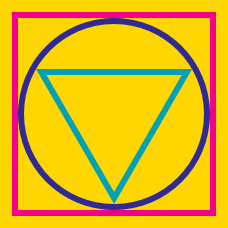Geometry

# Inscribed and Circumscribed Figures: Level 3 Challenges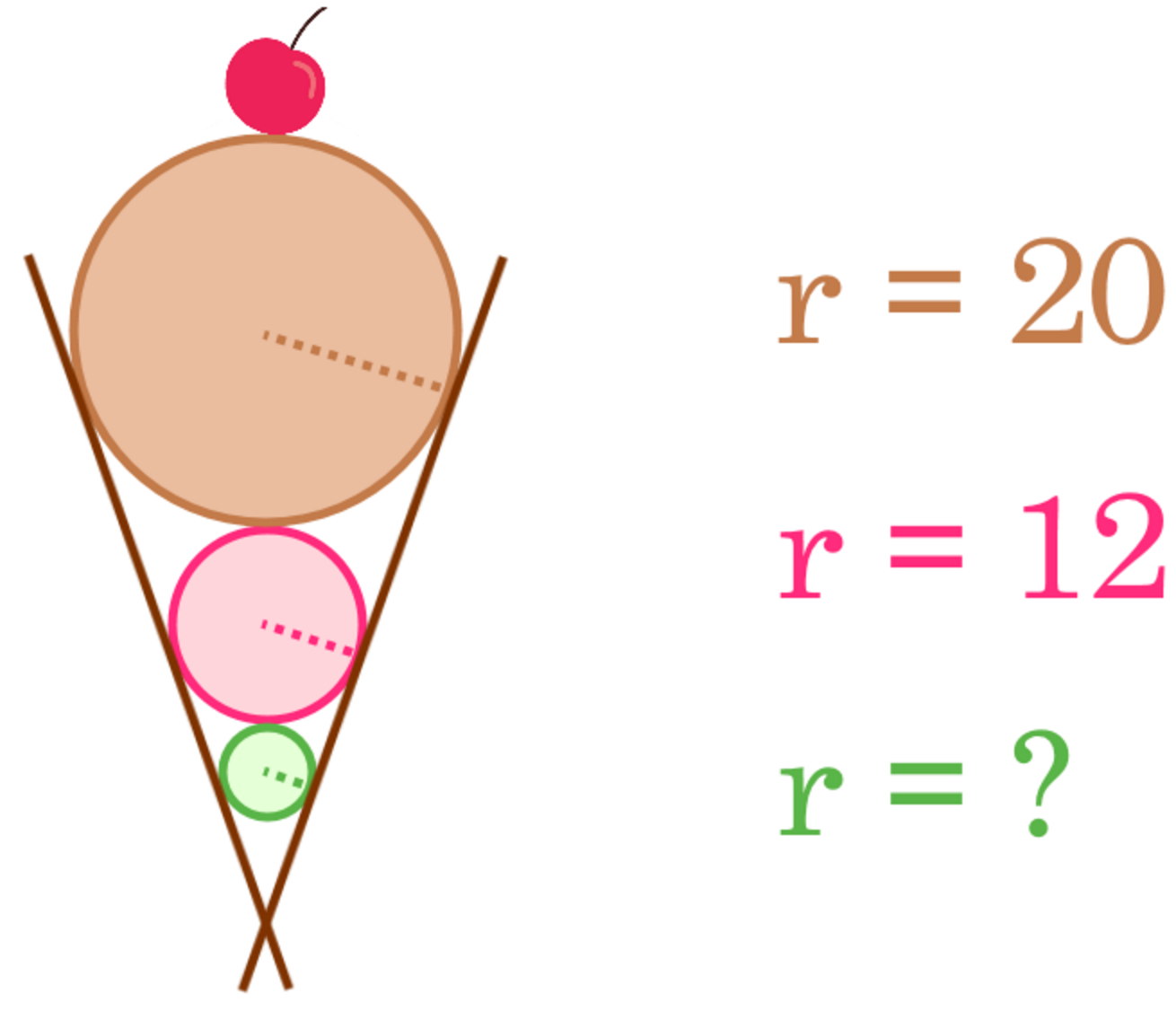Three balls are placed inside a cone such that each ball is in contact with the edge of the cone and the next ball. If the radii of the balls are 20 cm, 12 cm, and $r$ cm from top to bottom, what is the value of $r$?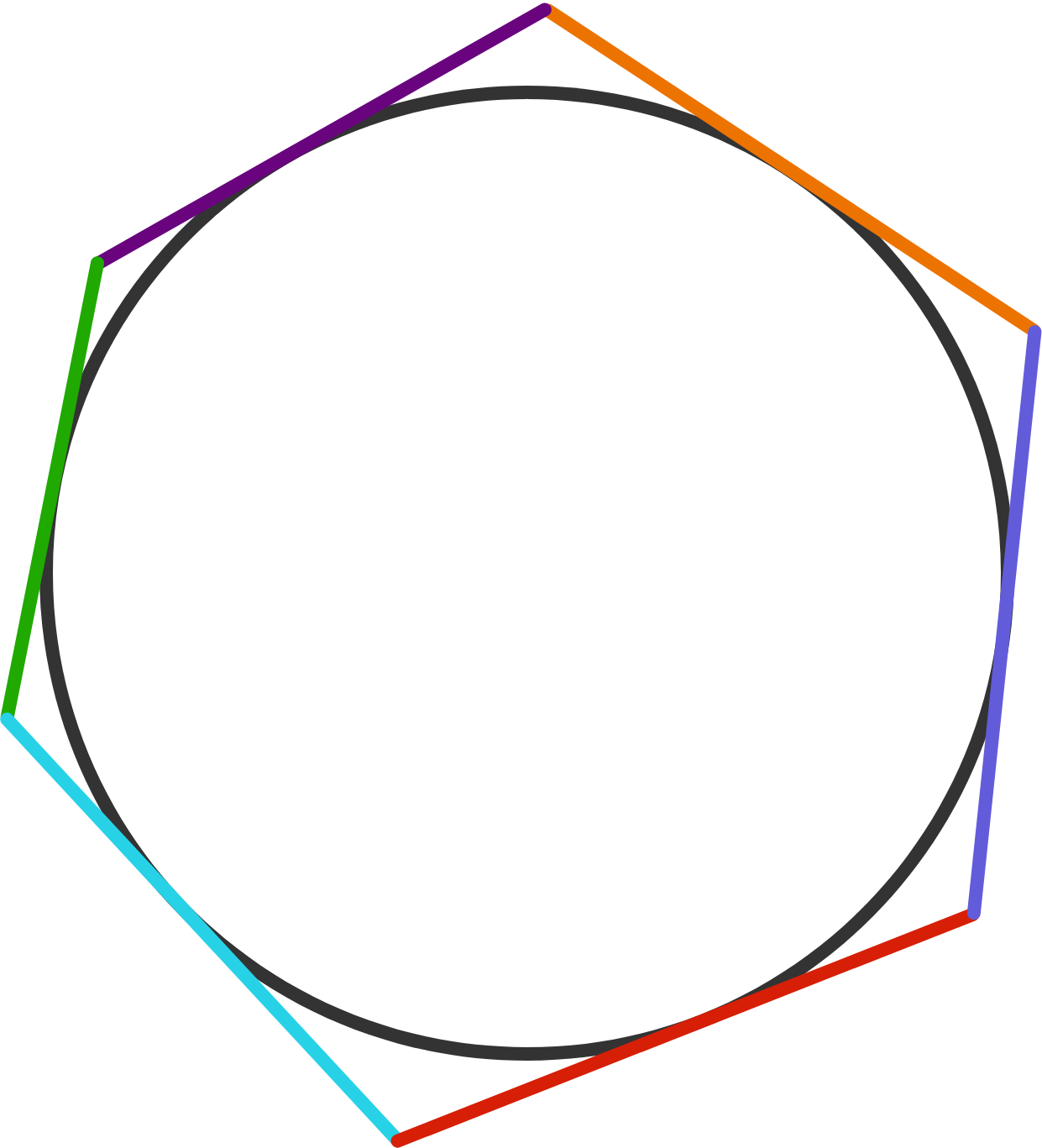A circle is inscribed in a hexagon, as shown in the diagram.

Is it possible that the side lengths of the hexagon are $7,9,11,13,15,17$ in some order?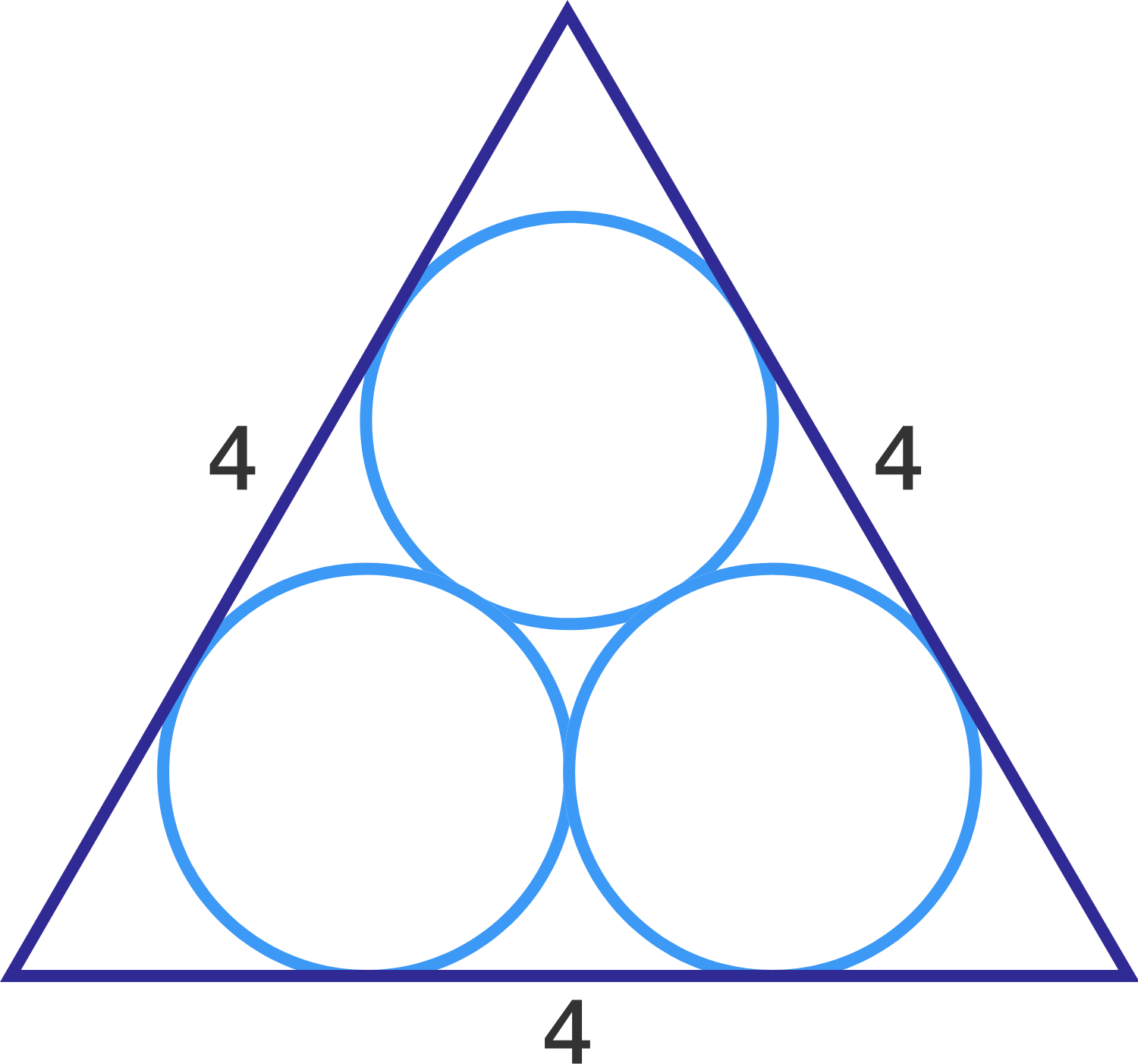In the figure, each of the three circles is tangent to the other two and each side of the equilateral triangle is tangent to two of the circles.

If the length of one side of the triangle is 4, what is the radius of each circle?

Details and assumptions:

$\bullet$ All the three circles have equal radii.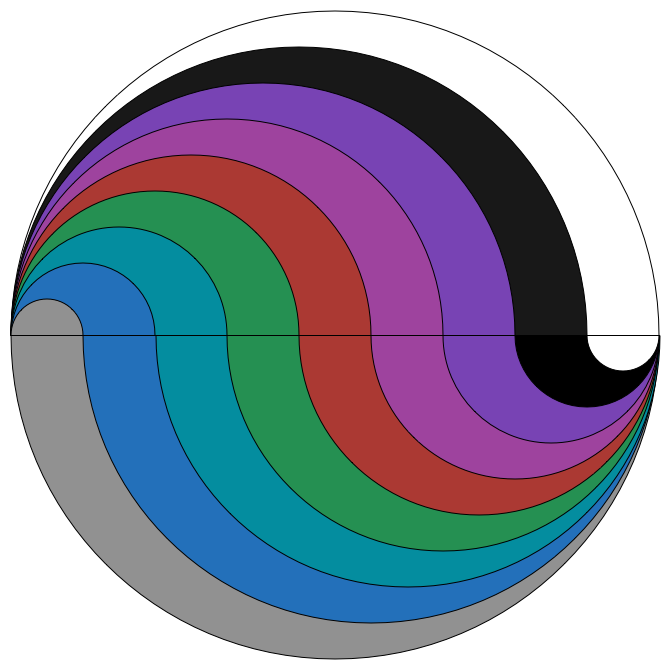The above is a circle of radius 9. It has been cut up into 9 different regions, using semicircles of integer radius.

The area of the largest region can be written as $A \pi$. What is $A$?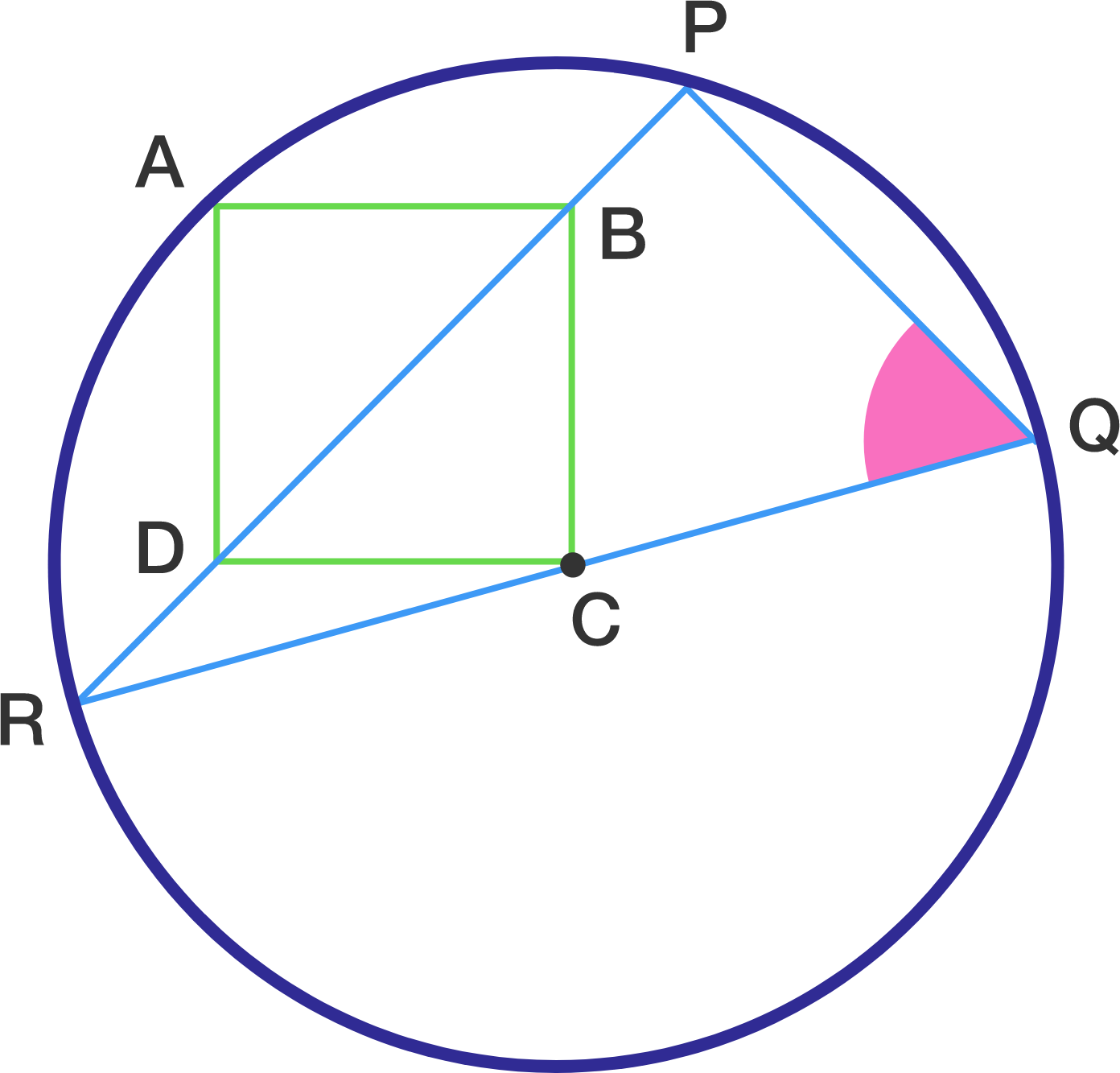Let $A,P,Q$ and $R$ points on the circumference with $C$ as center, $ABCD$ a square, $B$ and $D$ on $PR$, and $C$ on $QR$. Find $\angle PQR$ (in degrees).

×